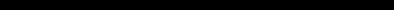# -SINCLAIR ZX SPECTRUM BASIC ProgrammingCHAPTER 8
Strings

#### Summary

Slicing. using TO. Note that this notation is not standard BASIC.

Given a string, a substring of it consists of some consecutive characters from it, taken in sequence. Thus "string" is a substring of "bigger string", but "b string" and "big reg" are not.

There is a notation called slicing for describing substrings, and this can be applied to arbitrary string expressions. The general form is

string expression (start TO finish)

so that, for instance,

"abcdef"(2 TO 5)="bcde"

If you omit the start, then 1 is assumed; if you omit the finish then the length of the string is assumed. Thus

"abcdef"( TO 5)="abcdef"(1 TO 5)="abcde"
"abcdef"(2 TO )="abcdef"(2 TO 6)="bcdef"
"abcdef"( TO )="abcdef"(1 TO 6)="abcdef"

(You can also write this last one as "abcdef"(), for what it's worth.)

A slightly different form misses out the TO and just has one number.

"abcdef"(3)="abcdef"(3 TO 3)="c"

Although normally both start and finish must refer to existing parts of the string, this rule is overridden by another one: if the start is more than the finish, then the result is the empty string. So

"abcdef"(5 TO 7)

gives error 3 subscript wrong because the string only contains 6 characters and 7 is too many, but

"abcdef"(8 TO 7)=""

and

"abcdef"(1 TO 0)=""

The start and finish must not be negative, or you get error B integer out of range. This next program is a simple one illustrating some of these rules.

10 LET a\$="abcdef"
20 FOR n=1 TO 6
30 PRINT a\$(n TO 6)
40 NEXT n
50 STOP

Type NEW when this program has been run and enter the next program:

10 LET a\$="ABLE WAS 1"
20 FOR n=1 TO 10
30 PRINT a\$(n TO 10),a\$((10-n) TO 10)
40 NEXT n
50 STOP

For string variables, we can not only extract substrings, but also assign to them. For instance, type

LET a\$="l'm the ZX Spectrum"

and then

LET a\$(5 TO 8)="******"

and

PRINT a\$

Notice how since the substring a\$(5 TO 8) is only 4 characters long, only the first four stars have been used. This is a characteristic of assigning to substrings: the substring has to be exactly the same length afterwards as it was before. To make sure this happens, the string that is being assigned to it is cut off on the right if it is too long, or filled out with spaces if it is too short - this is called Procrustean assignment after the inn-keeper Procrustes who used to make sure that his guests fitted the bed by either stretching them out on a rack or cutting their feet off.

If you now try

LET a\$()="Hello there"

and

PRINT a\$;"."

you will see that the same thing has happened again (this time with spaces put in) because a\$() counts as a substring.

LET a\$="Hello there"

will do it properly.

Complicated string expressions will need brackets round them before they can be sliced. For example,

"abc"+"def"(1 TO 2)="abcde"
["abc"+"def")(1 TO 2)="ab"

#### Exercise

 1 Try writing a program to print out the day of the week using string slicing. Hint: let the string be SunMonTuesWedThursFriSat.